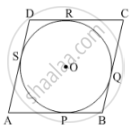# Prove that a Parallelogram Circumscribing a Circle is a Rhombus. - Mathematics

Sum

Prove that a parallelogram circumscribing a circle is a rhombus.

#### SolutionLet ABCD be the rhombus circumscribing the circle with centre O, such that AB, BC, CD and DA touch the circle at points P, Q, R and S respectively. We know that the tangents drawn to a circle from an exterior point are equal in length.

∴ AP = AS  ......(1)

BP = BQ  ......(2)

CR = CQ   ......(3)

DR = DS  ......(4)

Adding (1), (2), (3) and (4) we get

AP + BP + CR + DR = AS + BQ + CQ + DS

(AP + BP) + (CR + DR) = (AS + DS) + (BQ + CQ)

∴ AB + CD = AD + BC  .....(5)

Since AB = DC and AD = BC  .....(Opposite sides of parallelogram ABCD)

Putting in (5) we get, 2AB = 2AD

∴ AB = BC = DC = AD

Since a parallelogram with equal adjacent sides is a rhombus, ABCD is a rhombus.

Concept: Number of Tangents from a Point on a Circle
Is there an error in this question or solution?
2013-2014 (March) Delhi Set 1

Share